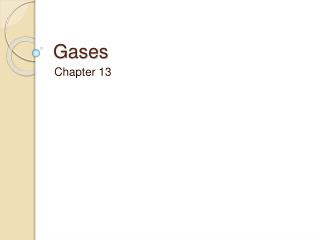DownloadDownload PresentationGases

# Gases

Télécharger la présentation## Gases

- - - - - - - - - - - - - - - - - - - - - - - - - - - E N D - - - - - - - - - - - - - - - - - - - - - - - - - - -
##### Presentation Transcript

1. Gases Chapter 13

2. Kinetic Molecular Theory • Gases consist of large numbers of tiny particles that are far apart relative to their size. • Collisions between gas particles and between particles and container walls are elastic collisions. • Gas particles are in continuous, rapid, random motion

3. 4) There are no forces of attraction or repulsion between gas particles. 5) The average kinetic energy of gas particles depends on the temperature of the gas. *This applies only to ideal gases

4. Diffusion-a spontaneous mixing of particles of two substances caused by their random motion. Ex: air freshener spreading throughout a room. Effusion-process by which gas particles pass through a tiny opening. Ex: filling your tire up with air with the little needle. Pressure-force per unit area

5. Honors Gas Examples

6. Avogadro’s Law Example 1 Consider two samples of nitrogen gas. Sample 1 contains 1.5mol of N2 and has a volume of 36.7L at 25°C and 1atm. Sample 2 has a volume of 16.5L at 25°C and 1atm. Calculate the number of moles of nitrogen in Sample 2.

8. V1=V2 n1 n2 n1=1.5mol 36.7 = 16.5 V1=36.7L 1.5 n V2=16.5L 36.7n=24.75 n2=? n=0.67mol T and P are constant so don’t have to include them in the formula/equation

9. Avogadro’s Law Example 2 If 2.55mol of helium gas occupies a volume of 59.5L at a particular temperature and pressure, what volume does 7.83 mol of helium occupy under the same conditions?

11. V1=V2 n1 n2 n1=2.55mol 59.5 = V V1=59.5L 2.55 7.83 n2=7.83mol 465.885=2.55V V2=? V=183L

12. Gas Stoichiometry Example 3 Calculate the volume of hydrogen produced at 1.50atm and 19°C by the reaction of 26.5g of zinc with excess hydrochloric acid according to the balanced equation. Zn + 2HClZnCl2 + H2

14. 65 72 135 2 Zn + 2HClZnCl2 + H2 26.5g 26.5g Zn x 1mol H2= 0.407692308mol H2 65g Zn V=? PV=nRT P=1.50atm (1.50)(V)=(0.40769)(0.082)(292) T=19C+273=292K 1.50V=9.76172936 V=6.5L

15. Example 4 Ammonia is commonly used as a fertilizer to provide a source of nitrogen for plants. A sample of ammonia occupies a volume of 5.00L at 25°C and 15.0atm. What volume will this sample occupy at STP?

17. V1=5.00L V2=? T1=25⁰C+273=298K T2=0⁰C+273=273K P1=15.0atm P2=1atm *STP stands for standard temperature and pressure 15.0(5.00)=1(V) 298 273 20475=298V 69L=V

18. Welcome to the Gas Phase to the tune of “Welcome to the Jungle” Guns and Roses 1987 cd#4

19. Verse 1 Welcome to the gas phase, it’s not all fun and games. PV=nRT, it’s enough to drive me insane. Laws of Boyle and Avogadro, and Charles, they would say. Measure volume and pressure, extrapolate to zero K.

20. Chorus Gas Phase, welcome to the gas phase, PV is n,n,n,n,n,n,n,n,R,T,T,T Use it along with stoichiometry.

21. Verse 2 Welcome to the gas phase, where Dalton’s law rules. Calculate partial pressure, mole fraction is the clue. Kinetic theory is applied, gives speed root-mean-square. Effusion and diffusion rates, make me pull my hair.

22. Chorus Gas Phase, welcome to the gas phase, PV is N,n,n,n,R,T Van der Waals’s, new equation, see.

23. P + a/Vm2, times Vm-b, is RT, for high P or low T